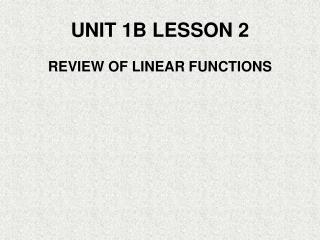Download PresentationUNIT 1B LESSON 2

# UNIT 1B LESSON 2 - PowerPoint PPT PresentationDownload Presentation## UNIT 1B LESSON 2

- - - - - - - - - - - - - - - - - - - - - - - - - - - E N D - - - - - - - - - - - - - - - - - - - - - - - - - - -
##### Presentation Transcript

1. UNIT 1B LESSON 2 REVIEW OF LINEAR FUNCTIONS

2. Equations of Lines The vertical line through the point (a, b) has equationx = asince everyx-coordinateon the line has the same valuea. Similarly, the horizontal line through (a, b) has equation y = b The horizontal line through thepoint(2, 3) has equation y = 3 The vertical line through thepoint(2, 3) has equation x = 2

3. Finding Equations of Vertical and Horizontal Lines EXAMPLE 1 Write the equations of the vertical and horizontal lines through the point Horizontal Line is y = 8 Vertical Line is x = –3

4. EXAMPLE 2: Reviewing Slope-Intercept Form of Linear Functions Y1 = 2x + 7 Slope y-intercept form y = mx + b slope y-intercept (0, b) y – intercept ( , )

5. y = 4xslope = m = _______ y -intercept ( , ) 3. 4. y = 3x – 5slope = m = _______ y -intercept ( , ) 6. slope = m = _______ y -intercept ( , ) = 6. slope = m = _______ y -intercept ( , ) Unit 1B Lesson 2 Page 1 EXAMPLES State the slopes and y-intercepts of the given linear functions. 4 0 , 0 3 0 ⅓ 0 , 0 ,

6. General Linear Equation Although the general linear form helps in the quick identification of lines, the slope-intercept form is the one to enter into a calculator for graphing. Ax + By = C By = – Ax + C y = – (A/B) x + C/B

7. Analyzing and Graphing a General Linear Equation Example 7 Find the slope and y-intercept of the line Rearrange for y y-intercept is Slope is

8. x + 2y = 3slope = m = _______ y -intercept ( , ) slope = m = _______ y -intercept ( , ) 8. 9. Unit 1B Lesson 2 Page 1 EXAMPLES State the slopes and y-intercepts of the given linear functions. 0 , 3/2 0 , 4/3

9. EXAMPLE 10 Find the equation in slope-intercept form for the line with slope and passes through the point Step 1: Solve for b using the point b = 7 Step 2: Find the equation

10. EXAMPLE 11 Find the equation in slope-intercept formfor the line parallel to and through the point (10, -1) Step 1: The slope of a parallel line will be Step 2: Solve for b using the point Step 3: Find the equation

11. EXAMPLE 12 Write the equation for the line through the point (– 1 , 2) that is parallelto the line L: y = 3x – 4 Step 1: Slope of L is 3 so slope of any parallel line is also 3. Step 2: Find b. Step 3: The equation of the line parallel to L: is Step 4: Graph on your calculator to check your work. Use a square window. Y1= 3x – 4 Y2= 3x + 5 (0, 5) (0, – 4)

12. EXAMPLE 13 Write the equation for the line that is perpendicular to and passes through the point (10, – 1 ) Step 1: The slope of a perpendicular line will be negative reciprocal Step 2: Solve for b using the point (10, – 1) Step 3: The equation of the line ┴to is Step 4: Graph on your calculator to check your work. Use a square window. Y1= Y2= –x+ 24

13. EXAMPLE 14 Write the equation for the line through the point (– 1, 2) that is perpendicularto the line L: y = 3x – 4 Step 1: Slope of L is 3 so slope of any perpendicular line is . • Step 2: Find b. Step 3: Find the equation of the line perpendicular to L: y = 3x – 4 Step 4: Graph on your calculator to check your work. Use a square window. Y1= 3x – 4 Y2

14. EXAMPLE 15 Find the equation in slope-intercept formfor the line that passes through the points (7, 2) and (5, 8). Step 1: Find the slope Step 2: Solve for b using eitherpoint Step 3: Find the equation (– 5, 8) (7, – 2)

15. EXAMPLE 16 Write the slope-intercept equation for the line through (– 2, –1) and (5, 4). Slope = m= (5, 4) (– 2, – 1) Equation for the line is

16. Finish the 5 questions in Lesson #2